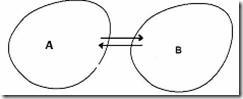# Engineering Mechanics | The three laws of motion

The Three Laws of Motion

The basis of all solutions to mechanics problems are the Newton’s laws of motion in one form or the other. The laws are:

First law: A body does not change its state of motion unless acted upon by a force. This law is based on observations but in addition it also defines an inertial frame . By definition an inertial frame is that in which a body does not change its state of motion unless acted upon by a force. For example to a very good approximation a frame fixed in a room is an inertial frame for motion of balls/ objects in that room. On the other hand if you are sitting in a train that is accelerating, you will see that objects outside are changing their speed without any apparent force. Then the motion of objects outside is changing without any force. The train is a non-inertial frame.

Second law: The second law is also part definition and part observation. It gives the force in terms of a quantity called the mass and the acceleration of a particle. It says that a force of magnitude F applied on a particle gives it an acceleration a proportional to the force. In other words

F = ma ,                                                       (1)

where m is identified as the inertial mass of the body. So if the same force – applied either by a spring stretched or compressed to the same length – acting on two different particles produces accelerations a1 and a2, we can say that

m1 a1 = m2 a2

Thus by comparing accelerations of a particle and of a standard mass (unit mass) when the same force is applied on each one them we get the mass of that particle. Thus gives us the definition of mass. It also gives us how to measure the force via the equation F = ma. One Newton (abbreviated as N) of force is that providing an acceleration of 1m/s2 to a standard mass of 1 kg. If you want to feel how much in 1 Newton , hold your palm horizontally and put a hundred gram weight on it; the force that you feel is about 1N.

Of course you cannot always measure the force applied by accelerating objects. For example if you are pushing a wall, how much force you are applying cannot be measured by observing the acceleration of the wall because the wall is not moving. However once we have adopted a measure of force, we can always measure it by comparing the force applied in some other situation.

In the first part of the course i.e. Statics we consider only equilibrium situations. We will therefore not be looking at F = ma but rather at the balance of different forces applied on a system. In the second part – Dynamics – we will be applying F = ma extensively.

Third Law: Newton’s third law states that if a body A applies a force F on body B , then Balso applies an equal and opposite force on A . (Forces do not cancel such other as they are acting on two different objects)Figure 1

Thus if they start from the position of rest A and B will tend to move in opposite directions. You may ask: if A and B are experiencing equal and opposite force, why do they not cancel each other? This is because – as stated above – the forces are acting on two different objects. We shall be using this law a lot both in static as well as in dynamics.

### 2 Responses to “Engineering Mechanics | The three laws of motion”

1.#### Anyewi George

Thanks, for posting professional items. i love my Mechanical Engineering profession.

2.